SAS/QC Graphics

# ODS Graphics

The following SAS/QC procedures support ODS Graphics:

• ANOM

• CAPABILITY

• CUSUM

• MACONTROL

• PARETO

• RELIABILITY

• SHEWHART

ODS Graphics provides the highest quality graphical output available from SAS/QC procedures. It is unaffected by global graphics statements, procedure options for controlling traditional graphics, and the GSTYLE system option. See Chapter 21, Statistical Graphics Using ODS (SAS/STAT 9.22 User's Guide), for a thorough discussion of ODS Graphics.

The following statements produce a histogram of the variable Thickness, which is discussed in the previous section. Here, the ODS GRAPHICS statement is specified to request ODS Graphics.

```ods listing style=statistical;
ods graphics on;
proc capability data=Trans noprint;
spec lsl = 3.45 usl = 3.55 cleft cright;
histogram Thickness;
run;
ods graphics off;
```

Figure 3.5 shows the ODS Graphics version of the histogram. Note that fonts, colors, and other attributes are determined by the STATISTICAL style. Options for specifying these attributes (as used, for example, in the statements that produced Figure 3.4) are not applicable with ODS Graphics and are ignored when you use the ODS GRAPHICS statement.

Figure 3.5 ODS Graphics Using STATISTICAL Style
Figure 3.6 through Figure 3.13 show examples of ODS Graphics output produced by SAS/QC procedures. These graphs were all created with the STATISTICAL style.

Figure 3.6 ANOM Chart
Figure 3.7 Box Chart (SHEWHART)
Figure 3.8 Comparative Histogram (CAPABILITY)
Figure 3.9 Moving Average Chart (MACONTROL)
Figure 3.10 Pareto Chart
Figure 3.11 Probability Plot (RELIABILITY)
Figure 3.12 Q-Q Plot (CAPABILITY)
Figure 3.13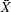and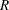Chart (SHEWHART)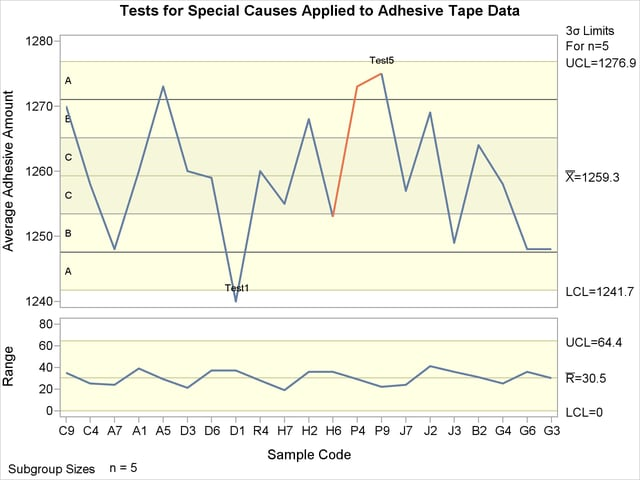Previous Page | Next Page | Top of Page